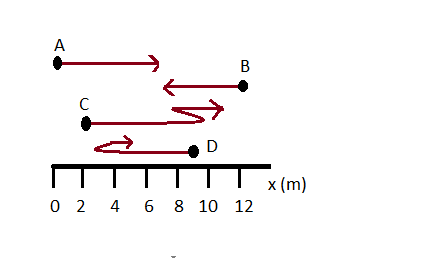# Find the following for path A in the figure below. (a) The total distance traveled. (b) The...

## Question:

Find the following for path A in the figure below.(a) The total distance traveled.

(b) The distance from start to finish.

(c) The displacement from start to finish.

## Distance and Displacement

Distance is defined as the total length or path covered between the initial and final points whereas the Displacement is defined as the straight line distance between the initial and final points.

(a)

For path A, distance is the length between the initial and final points.

{eq}\displaystyle X = 7 \ m {/eq}

(b)

Since the path is a straight line, therefore the total distance = 7 m

(c)

Since this is a straight line, therefore, the distance will be equal to displacement.

Hence Displacement will be 7 m.